# Modified Mukherjee-Brill prediction model of pressure gradient for multiphase flow in wells

Modified Mukherjee-Brill prediction model of pressure gradient for multiphase flow in wells

Yu Lei Ruiquan Liao Mengxia LiYong Li Wei Luo

School of Computer Science, Yangtze University, Jingzhou Hubei 434023, China

Gas Lift Innovation Center of CNPC, Shanshan Xinjiang 838200, China

Petroleum Engineering College, Yangtze University, Wuhan Hubei 430100, China

Gas Lift Innovation Center of CNPC, Wuhan Hubei 430100, China

The Branch of Key Laboratory of CNPC for Oil and Gas Production, Yangtze University, Wuhan Hubei 430100, China

School of Geosciences, Yangtze University, Wuhan Hubei 430100, China

Corresponding Author Email:
limengxia81@126.com
Page:
103-108
|
DOI:
https://doi.org/ 10.18280/ijht.350114
|
Accepted:
|
Published:
31 March 2017
| Citation

OPEN ACCESS

Abstract:

The Mukherjee-Brill prediction model of pressure gradient is the common calculation model in oil-gas field development. The laboratory experimental results of multiphase flow show that the average prediction relative error is 35% and the maximum relative error reaches 72%. By comparing the prediction residual of the Mukherjee-Brill model with the test condition parameters, this paper suggests that the predicted residual of Mukherjee-Brill model has a close relationship with the gas-liquid ratio and has no clear correlation with the water content of fluid when the volume flow rate of liquid is constant. This paper first builds a cubic regression model of the prediction residual of the Mukherjee-Brill model regarding the gas-liquid ratio, and subsequently, combining the cubic regression model with the Mukherjee-Brill model, builds a new prediction model of pressure gradient, the MBM model. The results for 90 test groups show that the average prediction relative error of the MBM model is reduced to 7.66%. The MBM model improves the prediction accuracy of the pressure gradient for multiphase flow, and assists in advancing the reliability of the design and analysis of oil and gas wells.

Keywords:

Multiphase Flow, Pressure Gradient, Prediction, Mukherjee-Brill Model, Regression Analysis

1. Introduction

The pressure gradient prediction for multiphase flow is an important theoretical foundation for the design and analysis of oil and gas wells. Much research has been carried out [1-5] in this field. The Mukherjee-Brill prediction model of pressure gradient  can be applied to wells with different inclination angles and it has been applied widely. This model is a representative calculation model of the pressure gradient. Reference  proposed a method based on the residual model to improve the existing prediction model of the gradient pressure and obtained good results. But the results of reference  have particularity and are difficult to define compared to other prediction models. The improved method proposed in reference  is generalized, but the implementation procedure is too complicated and affects the application of the improved model. Based on the results which are obtained from the experimental data in this paper, it can be shown that the average relative error between the pressure gradient calculated by the Mukherjee-Brill model and the one obtained from the experiment exceeds 35%. Thus it is necessary to further research the Mukherjee-Brill model and build a modified calculation model.

This paper firstly analyzes the prediction residual of the Mukherjee-Brill model, obtained by subtracting the experimental pressure gradient from the calculating pressure gradient by the Mukherjee-Brill model. The prediction residual is denoted as an MB residual, then analyzing the relationship between the MB residual and the gas-liquid ratio and building the cubic regression model with an independent variable GLR (gas-liquid ratio) and the dependent variable MB residual. The cubic regression model is denoted as PMB. Finally, a new prediction model of pressure gradient is constructed, called the MBM model. The calculation results show that the MBM model agrees with the experimental values more widely.

2. Prediction Performance of Mukherjee-Brill Model

The experimental conditions are given as follows. The liquid flow rates are 10 m3/d, 15 m3/d, 20 m3/d, 30 m3/d, 40 m3/d and 50 m3/d. The gas liquid ratios are 50, 100, 150, 200 and 300. The temperature is between 14 degrees Celsius and 17 degrees Celsius. The medium is air, white oil and water. The pressure gradient is between 0.55 kPa/m and 3.06 kPa/m. There are 90 groups of experimental data. The pipe diameter is 75 mm and the water contents are 30%, 60% and 90%. The roughness is taken as 0.0002 m. The process of experiment is similar to reference . The comparison between the pressure gradient calculated by the MB model and the experimental pressure gradient is shown in Figure 1. Most of the pressure gradient values calculated by the MB model are smaller than the experimental values. The average residual is 0.4945 kPa/m and the average relative error exceeds 35%.

## 1.jpgFigure 1. Comparison between the values calculated by MB model and experimental pressure gradient values under water content 30%

For different liquid flow rates, the curve with the abscissa GLR and the ordinate predicted residual is shown in Figure 2. From Figure 2, the following results can be observed: 1) among the residual curves with a water content of 30%, 60% and 90%, no one residual curve lies between any other two residual curves. It shows that the effect of water content on the residual has no obvious regulation; 2) with the increase of the liquid flow rate, the GLR corresponding to the maximum predicted residual decreases; 3) or the constant liquid flow rate, the residual curves have some similarities to their geometrical shapes. Hence, aiming at a constant liquid flow rate, this paper considers building a correlation between the predicted residual and the GLR.

## 21.jpg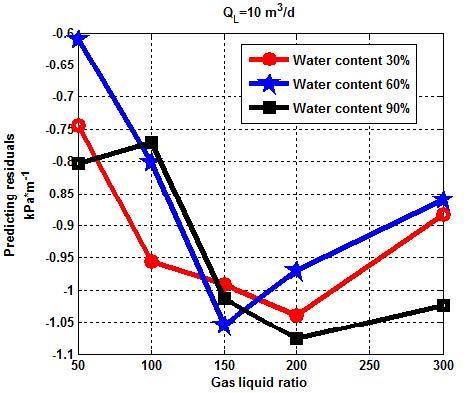## 22.jpg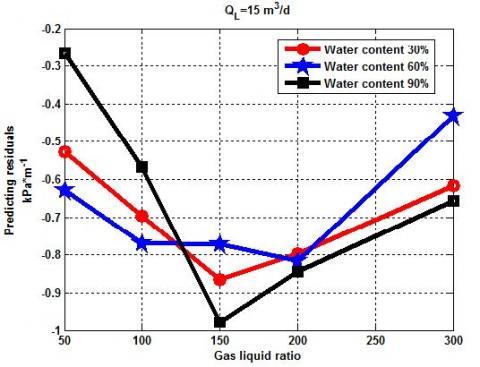## 23.jpg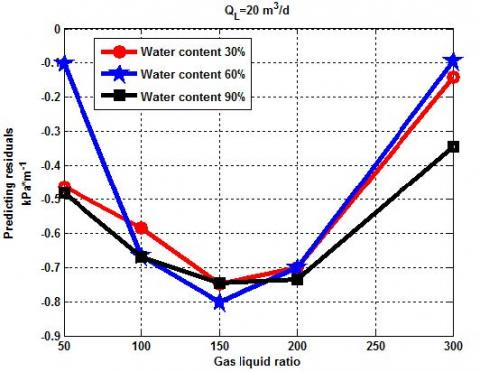## 24.jpg## 25.jpg## 26.jpgFigure 2. Relation between the predicted residual and GLR

3. Cubic Regression Model of Predicted Residual Of Mb Model

According to the above analysis, for six different liquid flow rates, cubic regression analysis for the predicted residual with respect to the GLR is required, since the difference in the order of magnitude between the MB predicted residual and the GLR is very large. Hence, the GLR is divided by 300 before building the cubic regression model such that the value ranges of the independent variable and dependent variable are close.

The cubic function model used in this paper is denoted as a PMB model. It is shown in Eq(1).

${{\hat{y}}_{i}}={{a}_{i}}{{\left( \frac{{{x}_{i}}}{300} \right)}^{3}}+b{{\left( \frac{{{x}_{i}}}{300} \right)}^{2}}+{{c}_{i}}\left( \frac{{{x}_{i}}}{300} \right)+{{d}_{i}}$, for $i=1,2,\cdots ,6$         (1)

In Eq(1), xi represents GLR with unit m3/m3; ${{\hat{y}}_{i}}$ is the regression value of predicted residual of MB model with unit kPa/m; ai, bi, ci and di are undetermined fitting coefficients.

Taking QL=10m3/d as an example which is shown in the first sub-graph of Figure 2, there are 15 data points (x1,j,y1,j) for j=1,2,3,…,15. The undetermined fitting coefficients ai, bi, ci and di can be determined by the following method.

Step 1 Build the objective function:

$E=\sum\limits_{j=1}^{15}{{{({{y}_{1,j}}-{{{\hat{y}}}_{1,j}})}^{2}}}=\sum\limits_{j=1}^{15}{{{[{{y}_{1,j}}-({{a}_{i}}{{\left( \frac{{{x}_{i}}}{300} \right)}^{3}}+b{{\left( \frac{{{x}_{i}}}{300} \right)}^{2}}+{{c}_{i}}\left( \frac{{{x}_{i}}}{300} \right)+{{d}_{i}})]}^{2}}}$          (2)

Step 2 Taking ai, bi, ci and di as unknown parameters, use the optimization algorithms [9-13] to minimize the objective function E. When the objective function E takes the minimum value, the corresponding values of ai, bi, ci and di  are the desired values.

Under different liquid flow rates, the values of ai, bi, ci and di are shown in Table 1.

Table 1. Values of ai, bi, ci and di  under different liquid flow rates

 Liquid flow rate (m3/d) ai, bi ci di 10 0.6687 0.0255 -1.0788 -0.5359 15 -0.3807 2.5795 -2.6759 -0.0893 20 -0.56 12 3.7839 -3.5583 0.1427 30 -9.3759 19.2185 -11.2864 1.4509 40 -9.9266 19.3181 -10.4263 1.2456 50 -8.9051 18.1804 -10.5071 1.5335

For the liquid flow rate 10 m3/d, 30 m3/d and 50 m3/d, MB residual and the regression value of residual are shown in Figure 3.

## 31.jpg## 32.jpg## 33.jpg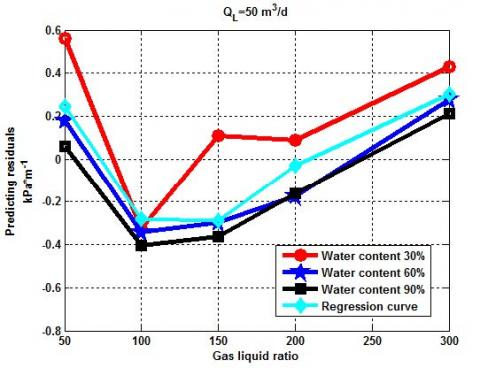Figure 3. Comparison between MB residual and the regression value of residual

4. Modified Mb Model

As described in the introduction，the MB residual is the difference value obtained by subtracting the experimental pressure gradient by the calculating pressure gradient of the MB model. Combining the MB model and the regression model of the residual, a prediction model of the pressure gradient can be built, called the MBM model in Eq(3).

MBM model=MB model-PMB model          (3)

The predicting pressure gradient of MBM model is

MBM Value=MB Value-PMB Value        (4)

where, MBM Value denotes the calculating pressure gradient value by the MBM model with unit kPa/m; MB Value denotes the calculating pressure gradient value by the MBM model with unit kPa/m; PMB Value denotes the regression value of residual by the residual model with unit kPa/m.

The relative error between the predicting pressure gradient by the MBM model and the experimental pressure gradient is defined as Eq(5).

err=|PMB Value -E Value|/E Value          (5)

In Eq(5), E Value denotes the experimental pressure gradient with unit kPa/m.

Based on the 90 groups of experimental data, the average value of the predicted relative error of the MBM model is 7.66% by comparing the relative error between the predicted pressure gradient of the MBM model and that of the MB model.

## 41.jpg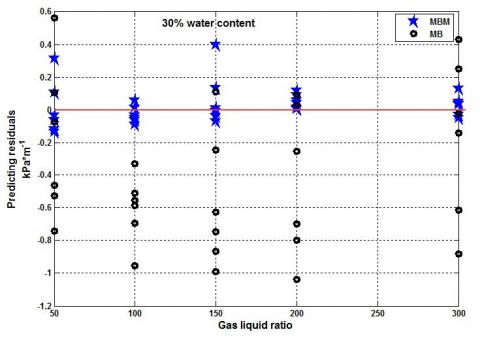## 42.jpg## 43.jpgFigure 4. Comparison between the predicting residual of MB model and that of MBM model

From Figure 4, it can be seen that the predicted residual of the MBM model is intensively distributed near the zero curve, which is the red axis in Figure 4. It shows that MBM model proposed in this paper has a better prediction effect.

5. Verification Of MBM Model

This paper uses the method described below to verify the MBM model. Firstly, it supposes that the regression model of the predicted residual corresponding liquid flow rate 15 m3/d is unknown. The regression models of predicted residual corresponding liquid flow rates 10 m3/d and 20 m3/d are considered as known conditions, and a linear interpolation is used to determine the predicted residual corresponding liquid flow rate of 15 m3/d. The specific form of the linear interpolation polynomial is shown in Eq(6).

rQ=(Q-10)/(20-10)r10+(20-Q)/(20-10)r20        (6)

In Eq(6), r represents the predicting residual and its subscript represents the corresponding liquid flow rate. When Q=15 m3/d, E Value is the predicting residual value corresponding to the liquid flow rate of 15 m3/d. The verification results are shown in Table 2.

Table 2. Verification results of MBM model under liquid volume flow rate 15 m3/d

 GLR Water content Relative error of MB model(%) Relative error calculated by Eq(1) (%) Relative error calculated by Eq(6) (%) 50 30% 20.91 2.42 0.15 60% 23.85 6.15 3.70 90% 11.5 8.48 11.25 100 30% 36.45 0.71 3.18 60% 42.54 3.35 0.75 90% 34.73 8.59 11.46 150 30% 53.93 2.26 0.42 60% 48.49 3.73 6.43 90% 58.43 8.83 6.26 200 30% 59.31 3.18 6.17 60% 59.28 1.80 4.73 90% 62.03 0.37 2.58 300 30% 63.03 5.15 6.15 60% 55.01 17.24 15.99 90% 64.44 8.92 9.87 Average value(%) 46.26 5.41 5.94

From Table 2, it can be seen that for the liquid flow rate 15 m3/d, the average relative error of the predicted pressure gradient is 5.41% directly calculated by Eq (1) and 5.94% calculated by Eq (6) which uses the interpolation. Both results significantly improve the average relative error of the original MB model which is 46.26%. It shows that the modified model is feasible and has a better predictive effect.

6. Conclusions

(1) This paper builds a cubic regression model of the predicted residual of the Mukherjee-Brill model regarding the gas-liquid ratio, and subsequently combining the cubic regression model with the Mukherjee-Brill model, builds a new prediction model of pressure gradient, the MBM model.

(2) For the pressure gradient under the experimental conditions, the average value of the predicting relative error of MBM model is 7.66%

(3) The verification results based on the interpolation show the effectiveness of the modified model proposed in this paper.

(4) The MBM model proposed in this paper is more concise compared to reference  and has better extendibility compared to reference .

Acknowledgment

The authors will thank people in the Branch of Key Laboratory of CNPC for Oil and Gas Production and Key Laboratory of Exploration Technologies for Oil and Gas Resources for their great help. This paper is supported by National Natural Science Foundation of China (61572084 and 51504038).

Nomenclature
 a undetermined fitting coefficient b undetermined fitting coefficient c undetermined fitting coefficient d undetermined fitting coefficient E residual function err relative error between the predicting pressure gradient by MBM model and the experimental pressure gradient is defined as Eq(5) E Value experimental pressure gradient, kPa/m MB Mukherjee-Brill model MBM new prediction model of pressure gradient MBM Value pressure gradient value by MBM model, kPa/m MBValue calculating pressure gradient value by MBM model, kPa/m PMB cubic regression model PMBValue regression value of residual by the residual model, kPa/m Q flow rate, m3/d r predicting residual x gas liquid ratio, m3/m3 y regression value of predicted residual of MB model, kPa/m (x1,j,y1,j) data point Subscripts i number of values of liquid flow rate j number of data points L liqud phase
References

 Zhou R.L., Zhou J., Luo Y., Li X., Liu Y.X. (2013). Research and application of liquid-carrying model for low permeability and water production gas reservoir, Lithologic Reservoirs, Vol. 4, No. 4, pp. 123-128. DOI: 10.3969/j.issn.1673-8926.2013.04.024

 Tian X.L., Jiang H.Y., Yue Y.R., Zhang Y.C. (2013). Research on production law for water drainage and gas production by gas lift, Fault-Block Oil & Gas Field, Vol. 19, No. 6, pp. 760-763. DOI: 10.6056/dkyqt201206020

 Bai X.H., Tian W., Tian S.B., Li K. (2014). Optimization on wellbore flow parameters of gas lift deliquification in low production and liquid loading gas wells, Fault-Block Oil & Gas Field, Vol. 21, No. 1, pp. 125–128. DOI: 10.6056/dkyqt201401031

 Tian Y., Wang Z.B., Li Y.C., Bai H.F., Li K.Z. (2015).         Evaluation and optimization of wellbore pressure drop model for drainage and gas recovery by velocity string, Fault-Block Oil & Gas Field, Vol. 22, No. 1, pp. 130-133. DOI: 10.6056/dkyqt201501029

 Ahmed M.M., Ayoub M.A. (2014). A Comprehensive Study on the Current Pressure Drop Calculation in Multiphase Vertical Wells; Current Trends and Future Prospective, Journal of Applied Sciences, Vol. 14, No. 21, pp. 3162–3171. DOI: 10.3923/jas.2014.3162.3171

 Mukherjee H., Brill J.P. (1983). Liquid Holdup Correlations for Inclined Two-Phase Flow, Journal of Petroleum Technology, Vol. 35, No. 5, pp. 1003 – 1008. DOI: http://dx.doi.org/10.2118/10923-PA

 Li M.X., Liao R.Q., Dong Y., Luo W. (2016). New prediction method of pressure drop for oil-gas-water three-phase vertical flow, Journal of the Balkan Tribological Association, Vol. 22, No. 1A-I, pp. 680-695.

 Dong Y., Li M.X., Liao R.Q., Luo W. (2016). Modification of Beggs-Brill pressure gradient predicting model for multiphase flow in vertical wells, Journal of Oil and Gas Technology, Vol. 38, No. 1, pp. 40-47. DOI: 10.12677/jogt.2016.11006

 Dong Y., Wu C.S., Guo H.M. (2015). Particle swarm optimization algorithm with adaptive chaos perturbation, Cybernetics and Information Technologies, Vol. 15, No. 6, pp. 70-80. DOI: 10.1515/cait-2015-0068

 Dong Y., Guo H.M. (2011). Adaptive chaos particle swarm optimization based on colony fitness variance, Application Research of Computers, Vol. 28, No. 3, pp. 854-856. DOI: 10.3969/j.issn.1001-3695.2011.03.015

 Li M.X., Liao R.Q. (2015). A new chaos particle swarm optimization combining the chaotic perturbation, International Journal of Signal Processing, Image Processing and Pattern Recognition, Vol. 8, No. 4, pp. 41-48. DOI: 10.14257/ijsip.2015.8.4.04

 Bounaouara H., Ettouati H., Ticha H.B., Mhimid A., Sautet J.C. (2015). Numerical simulation of gas-particles two phase flow in pipe of complex geometry: pneumatic conveying of olive cake particles toward a dust burner, International Journal of Heat and Technology, Vol. 33, No. 2, pp. 99-106. DOI: 10.18280/ijht.330114

 Lin T., Wu P., Gao F.M., Yu Y, Wang L.H. (2015). Study on SVM temperature compensation of liquid ammonia volumetric flowmeter based on variable weight PSO, International Journal of Heat and Technology, Vol. 33, No. 2, pp. 151-156. DOI: 10.18280/ijht.330224